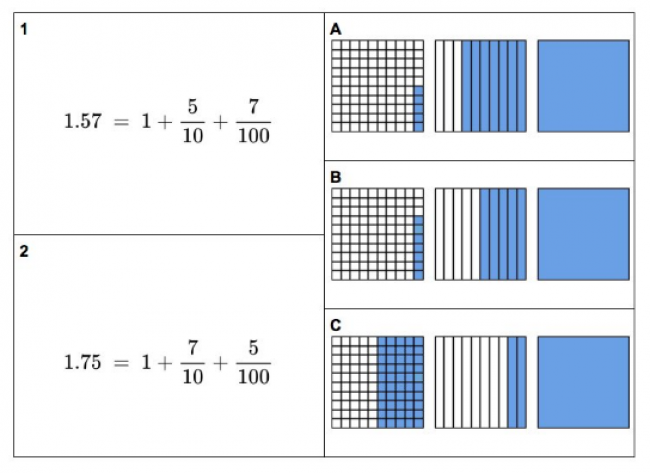# Fractions and Decimals

Preview:This task allows students to see how fractions and decimals can both be represented using similar visuals.

Connecting Representations
Big Idea:
Grade 4: Fractions and decimals are types of numbers that can represent quantities.
Content:
Fractions and decimals are numbers that represent an amount or quantity. Fractions and decimals can represent parts of a region, set, or linear model. Fractional parts and decimals are equal shares or equal-sized portions of a whole or unit. understanding the relationship between fractions and decimals Place value: 1000s, 100s, 10s, and 1s understanding the relationship between digit places and their value, to 10 000
Curricular Competency:
Reasoning and analyzing: Develop mental math strategies and abilities to make sense of quantities
Understanding and solving: Develop, demonstrate, and apply mathematical understanding through play, inquiry, and problem solving
Understanding and solving: Visualize to explore mathematical concepts
Understanding and solving: Develop and use multiple strategies to engage in problem solving
Communicating and representing: Communicate mathematical thinking in many ways
Communicating and representing: Use mathematical vocabulary and language to contribute to mathematical discussions
Communicating and representing: Explain and justify mathematical ideas and decisions
Communicating and representing: Represent mathematical ideas in concrete, pictorial, and symbolic forms
Connecting and reflecting: Reflect on mathematical thinking